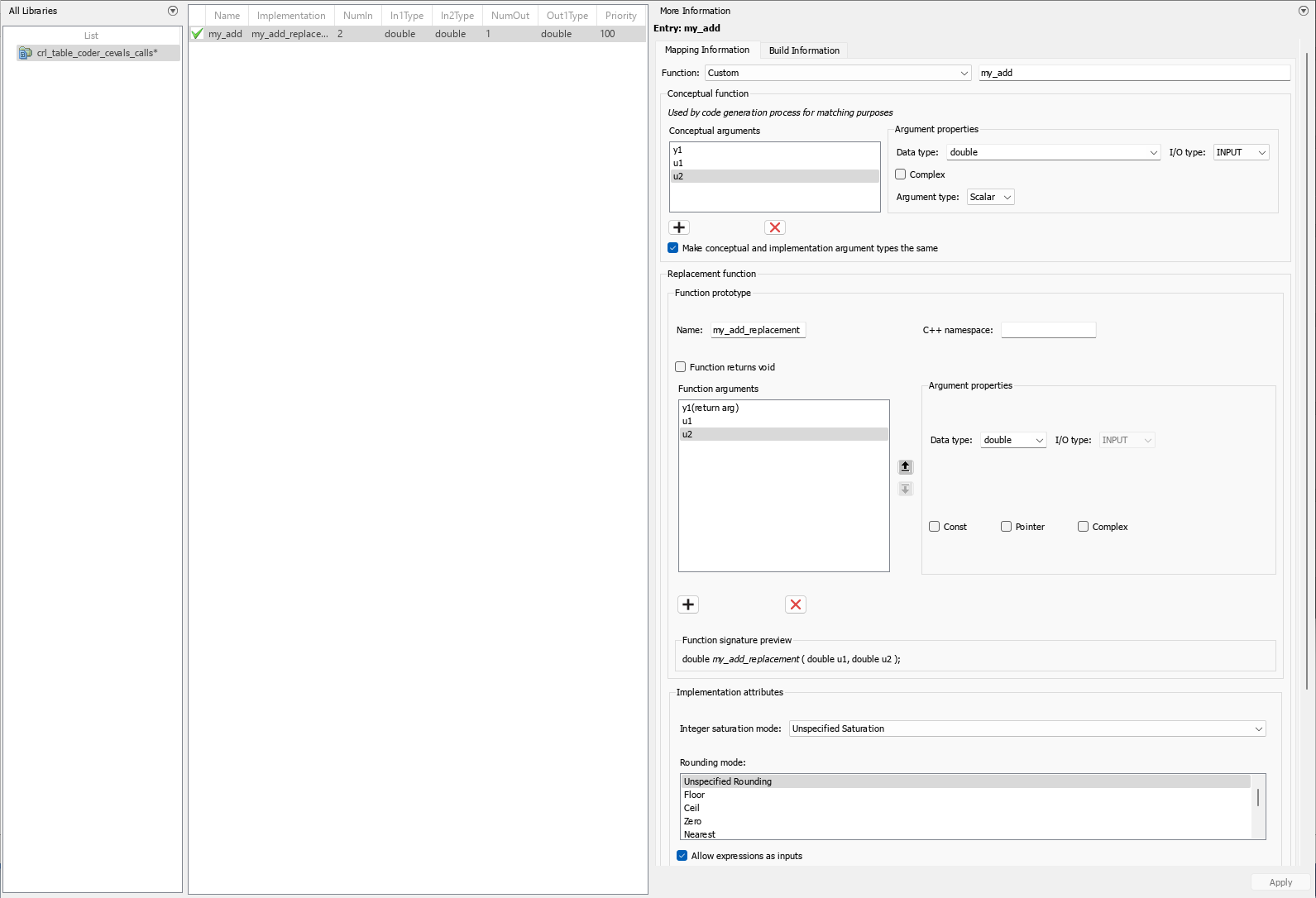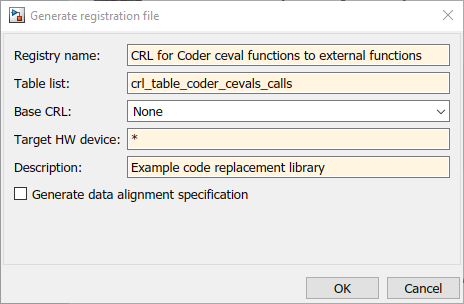## Replace `coder.ceval` Calls to External Functions

The `coder.ceval` function calls external C/C++ functions from code generated from MATLAB® code. The code replacement software supports replacement of the function that you specify in a call to `coder.ceval`. An application of this code replacement scenario is to write generic MATLAB code that you can customize for different platforms with code replacements. A code replacement library can define hardware-specific code replacements for the function call. Use `coder.ceval` in MATLAB code from which you want to generate C code using:

• MATLAB Coder™

• MATLAB code in a Simulink® MATLAB Function block

To develop a code replacement library use either the interactive or programmatic approach. For more information, see Develop a Code Replacement Library.

### Example Files

For the examples in Interactively Develop a Code Replacement Library and Programmatically Develop a Code Replacement Library you must have set up the following:

• Custom C function `my_add.c`.

```/* my_add.c */ #include "my_add.h" double my_add(double in1, double in2) { return in1 + in2; }```
• Custom C header file `my_add.h`.

```/* my_add.h */ double my_add(double in1, double in2); ```
• MATLAB function `call_my_add.m`, which uses `coder.ceval` to invoke `my_add.c`.

```function y = call_my_add(in1, in2) %#codegen y=0.0; if ~coder.target('Rtw') % Executing in MATLAB, call MATLAB equivalent of C function my_add y= in1+in2; else % Executing in generated code, call C function my_add y = coder.ceval('my_add', in1, in2); end ```
• MATLAB test function `call_my_add_test.m`, which calls `call_my_add.m`.

```in1=10; in2=20; y = call_my_add(in1, in2); disp('Output') disp('y =') disp(y);```
• Replacement C function `my_add_replacement.c`.

```/* my_add_replacement.c */ #include "my_add_replacement.h" double my_add_replacement(double in1, double in2) { return in1 + in2; }```
• Replacement C header file `my_add_replacement.h`.

```/* my_add_replacement.h */ double my_add_replacement(double in1, double in2); ```

### Interactively Develop a Code Replacement Library

1. Open the Code Replacement Tool (crtool), from the MATLAB command line with the following command:

`>>crtool`
2. Create a table.

1. From the crtool menu, select File > New Table.

2. In the right pane, name the table `crl_table_coder_cevals_calls`. Click Apply.

3. Create an entry. From the crtool menu, select File > New entry > Function.

4. Create entry parameters. In the Function drop-down list, select `custom`. Function information appears in the crtool. For this example, specify the parameter as `my_add` .

5. Create the conceptual representation. The conceptual representation describes the signature of the function that you want to replace. In the Conceptual function subsection of the crtool, specify the return argument, `y1`, and the input arguments, `u1` and `u2` with the Data Type of double and the Argument Type of Scalar.

6. Create the implementation representation. The implementation representation describes the signature of the optimization function. For this example, to specify that the implementation arguments have the same order and properties as the conceptual arguments, select the Make conceptual and implementation argument types the same check box.

Specify a Name for the replacement function under Function prototype.

7. Specify build information. Click the Build Information tab to open the build requirements pane. Specify the files (source, header, object) that the code generator requires for code replacement. For this example, set the Implementation header file to `my_add_replacement.h`.

8. Validate and save the table. Click the Mapping Information tab and verify the fields are filled in as shown. Click Validate entry. In the crtool menu, select File > Save table > Save.9. Register a code replacement library. Registration creates a library composed of the tables that you specify. Select File > Generate registration file. In the Generate registration file dialog box, fill out these fields:To use your code replacement library, refresh your current MATLAB session with the command:

`>>sl_refresh_customizations`

10. Verify the code replacement library. From the MATLAB command line, open the library by using the Code Replacement Viewer and verify that the table and entry are correctly specified. For more information, see Verify Code Replacement Library. Configure your model to use the code replacement library, generate code, and verify that replacement occurs as expected. If unexpected behavior occurs, examine the hit and miss logs to troubleshoot the issues.

### Programmatically Develop a Code Replacement Library

1. Open the programmatic interface from the MATLAB menu by selecting New > Function.

2. Create a table.

1. Create a function with the name of your code replacement library table that does not have arguments and returns a table object. You can use this function to call your code replacement library table.

2. Create a table object by calling `RTW.TflTable`.

```function hTable = crl_table_coder_cevals_calls() % Create a function to call the code replacement library table %% Create a table object hTable = RTW.TflTable; ```
3. Create an entry. Because this example replaces a function, create a code replacement entry in your table by calling the entry function `RTW.TflCFunctionEntry`.

```function hTable = crl_table_coder_cevals_calls() % Create a code replacement library table %% Create a table object hTable = RTW.TflTable; %% Create an entry hEntry = RTW.TflCFunctionEntry; ```
4. Create entry parameters. Because this examples replaces a function, create entry parameters by calling the function `setTflCFunctionEntryParameters`.

```function hTable = crl_table_coder_cevals_calls() % Create a code replacement library table %% Create a table object hTable = RTW.TflTable; %% Create an entry hEntry = RTW.TflCFunctionEntry; %% Create entry parameters hEntry.setTflCFunctionEntryParameters( ... 'Key', 'my_add', ... 'Priority', 100, ... 'ImplementationName', 'my_add_replacement', ... 'ImplementationHeaderFile', 'my_add_replacement.h', ... 'ImplementationSourceFile', 'my_add_replacement.c');```
5. Create the conceptual representation. The conceptual representation describes the signature of the function that you want to replace. To explicitly specify argument properties, call the function `getTflArgFromString`.

```function hTable = crl_table_coder_cevals_calls() % Create a code replacement library table %% Create a table object hTable = RTW.TflTable; %% Create an entry hEntry = RTW.TflCFunctionEntry; %% Create entry parameters hEntry.setTflCFunctionEntryParameters( ... 'Key', 'my_add', ... 'Priority', 100, ... 'ImplementationName', 'my_add_replacement', ... 'ImplementationHeaderFile', 'my_add_replacement.h', ... 'ImplementationSourceFile', 'my_add_replacement.c'); %% Create the conceptual representation arg = hEntry.getTflArgFromString('y1','double'); arg.IOType = 'RTW_IO_OUTPUT'; hEntry.addConceptualArg(arg); arg = hEntry.getTflArgFromString('u1','double'); hEntry.addConceptualArg(arg); arg = hEntry.getTflArgFromString('u2','double'); hEntry.addConceptualArg(arg); ```
6. Create the implementation representation. The implementation representation describes the signature of the optimization function. To specify that the implementation arguments have the same order and properties as the conceptual arguments, call the function `getTflArgFromString`.

```function hTable = crl_table_coder_cevals_calls() % Create a code replacement library table %% Create a table object hTable = RTW.TflTable; %% Create an entry hEntry = RTW.TflCFunctionEntry; %% Create entry parameters hEntry.setTflCFunctionEntryParameters( ... 'Key', 'my_add', ... 'Priority', 100, ... 'ImplementationName', 'my_add_replacement', ... 'ImplementationHeaderFile', 'my_add_replacement.h', ... 'ImplementationSourceFile', 'my_add_replacement.c'); %% Create the conceptual representation arg = hEntry.getTflArgFromString('y1','double'); arg.IOType = 'RTW_IO_OUTPUT'; hEntry.addConceptualArg(arg); arg = hEntry.getTflArgFromString('u1','double'); hEntry.addConceptualArg(arg); arg = hEntry.getTflArgFromString('u2','double'); hEntry.addConceptualArg(arg); %% Create the implementation representation arg = hEntry.getTflArgFromString('y1','double'); arg.IOType = 'RTW_IO_OUTPUT'; hEntry.Implementation.setReturn(arg); arg = hEntry.getTflArgFromString('u1','double'); hEntry.Implementation.addArgument(arg); arg = hEntry.getTflArgFromString('u2','double'); hEntry.Implementation.addArgument(arg); %% Add the entry to the table hTable.addEntry(hEntry); ```
7. Specify build information. In the entry parameters, specify files (header, source, object) that the code generator needs for code replacement. For this example, build information is not required.

8. Validate and save the customization file. From the MATLAB menu, save this customization file by selecting File > Save. From the command line, validate the code replacement library table by calling it:

`>> hTable = crl_table_coder_cevals_calls`
9. Register the code replacement library. Registration creates a code replacement library by defining the library name, code replacement tables, and other information. Create a registration file by using these specifications:

```function rtwTargetInfo(cm) cm.registerTargetInfo(@loc_register_crl); end function this = loc_register_crl this(1) = RTW.TflRegistry; this(1).Name = 'CRL for coder.ceval calls to external functions code replacement’; this(1).TableList = {' crl_table_coder_cevals_calls.m'}; % table created in this example this(1).TargetHWDeviceType = {'*'}; this(1).Description = 'Example code replacement library'; end ```

To use your code replacement library, refresh your current MATLAB session with the command:

`>>sl_refresh_customizations`

10. Verify the code replacement library. From the MATLAB command line, open the library by using the Code Replacement Viewer and verify that the table and entry are correctly specified. For more information, see Verify Code Replacement Library. Configure your model to use the code replacement library, generate code, and verify that replacement occurs as expected. If unexpected behavior occurs, examine the hit and miss logs to troubleshoot the issues.